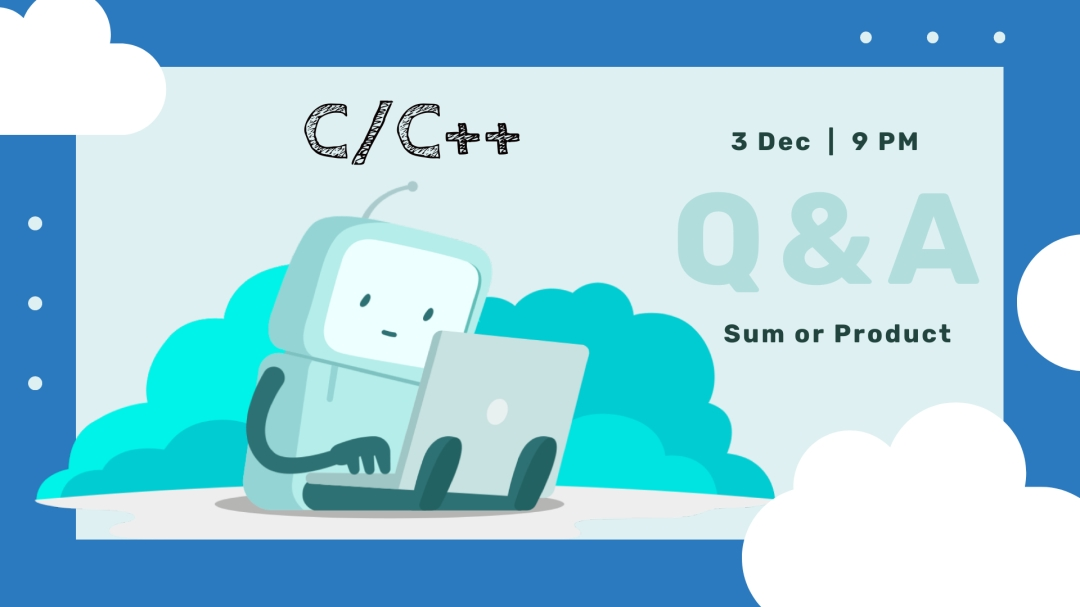# C++/C Solution (Sum or Product) - Write a program that asks the user for a number N and a choice C. And then give them the possibility to choose between computing the sum and computing the product of all integers in the range 1 to N (both inclusive).C++/C Solution (Sum or Product)

## Question :

Write a program that asks the user for a number N and a choice C. And then give them the possibility to choose between computing the sum and computing the product of all integers in the range 1 to N (both inclusive).
If C is equal to -
1. then print the sum
2. then print the product
*Any other number, then print '-1' (without the quotes)*

Input format :
Line 1 : Integer N
Line 2 : Choice C

Output Format :
Sum or product according to user's choice

Constraints :
1 <= N <= 12

Sample Input 1 :
10
1

Sample Output 1 :
55

Sample Input 2 :
10
2

Sample Output 2 :
3628800

Sample Input 3 :
10
4

Sample Output 3 :
-1

Solution Code
#include <iostream>
using namespace std;

int main () {
int sum = 0, prod = 1;
int num;
// cout << "Enter a Number ?" << endl;
cin >> num;
int choice;
// cout << "Do you want to compute the sum or product?" << endl;
cin >> choice;

if ( choice == 1 ) {
for ( int i = 1; i <= num; i++) {
sum += i;
}
cout << sum << endl;
}
else if( choice == 2 ) {
for ( int i = 1; i <= num; i++) {
prod = prod * i;
}
cout << prod << endl;
}
else {
cout << -1 << endl;
}
}
Thankyou Keep Visiting for More Coding Problems :D)
Show Comment Box
Hide Comment Box